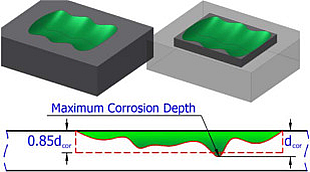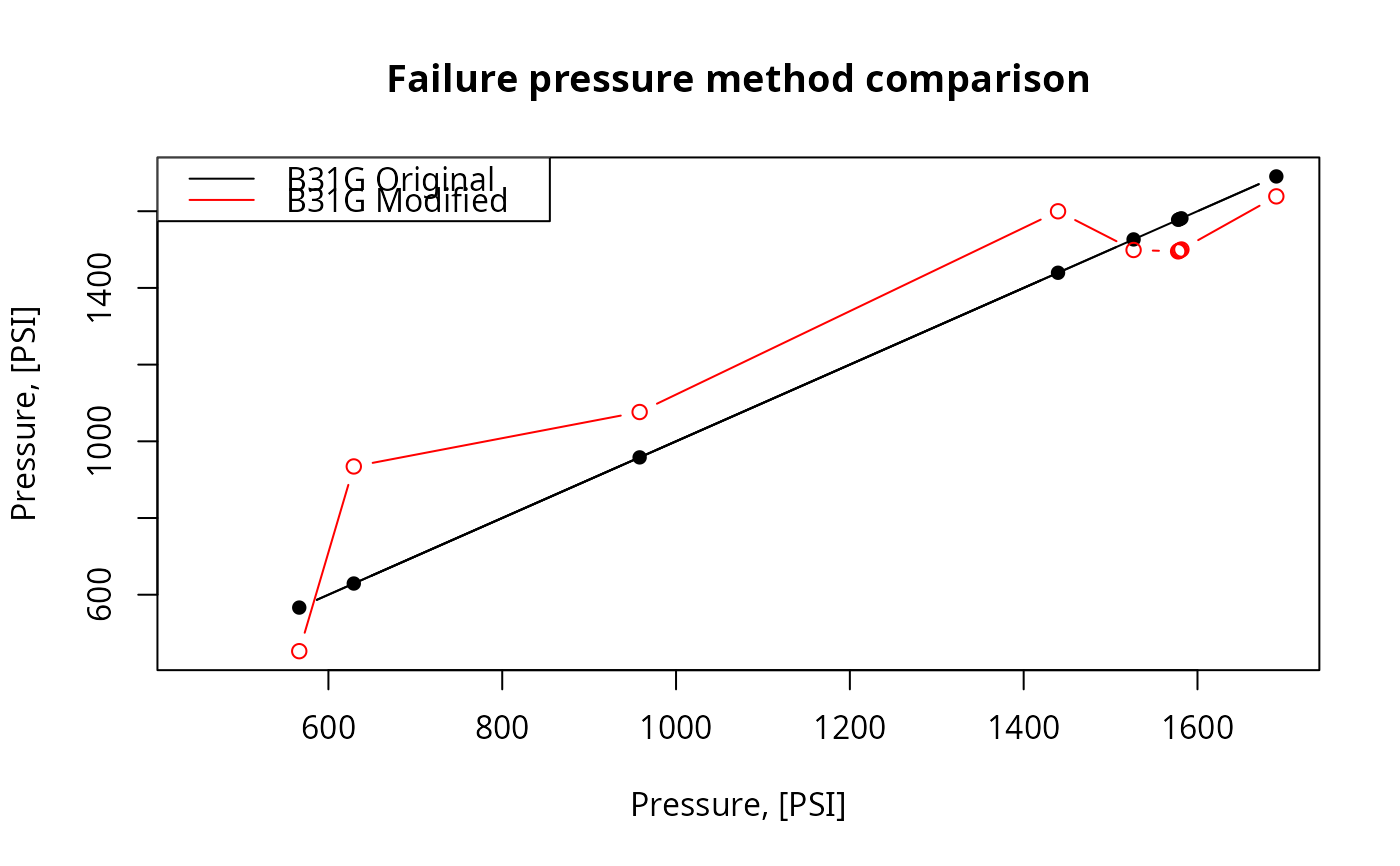Calculate failure pressure of the corroded pipe according to Modified B31G, Level-1 algorithm listed in ASME B31G-2012.

The next assumption of the corrosion shape is adopted by Modified B31G:There dcor represents argument depth.

b31gmodpf(d, wth, smys, depth, l)

## Arguments

d

nominal outside diameter of the pipe, [inch]. Type: assert_double.

wth

nominal wall thickness of the pipe, [inch]. Type: assert_double.

smys

specified minimum yield of stress (SMYS) as a characteristics of steel strength, [PSI]. Type: assert_double.

depth

measured maximum depth of the corroded area, [inch]. Type: assert_double.

l

measured maximum longitudinal length of corroded area, [inch]. Type: assert_double.

## Value

Estimated failure pressure of the corroded pipe, [PSI]. Type: assert_double.

## Details

Since the definition of flow stress, Sflow, in ASME B31G-2012 is recommended with Level 1 as follows:

$$Sflow = 1.1SMYS$$

no other possibilities of its evaluation are incorporated.

For this code we avoid possible semantic optimization to preserve readability and correlation with original text description in ASME B31G-2012. At the same time source code for estimated failure pressure preserves maximum affinity with its semantic description in ASME B31G-2012.

Numeric NAs may appear in case prescribed conditions of use are offended.

Other fail pressure functions: b31gpf, dnvpf, shell92pf, pcorrcpf

Other ASME B31G functions: b31crvl(), b31gacd(), b31gacl(), b31gafr(), b31gdep(), b31gops(), b31gpf(), b31gsap()

## Examples

 ## Example: maximum percentage disparity of original B31G
## algorithm and modified B31G showed on CRVL.BAS data
with(b31gdata, {
original  <- b31gpf(d, wth, smys, depth, l)
modified  <- b31gmodpf(d, wth, smys, depth, l)
round(max(100*abs(1 - original/modified), na.rm = TRUE), 4)
})
#>  32.6666
## Output:
# 32.6666

## Example: plot disparity of original B31G algorithm and
## modified B31G showed on CRVL data
with(b31gdata[-(6:7),], {
b31g  <- b31gpf(depth, wth, smys, depth, l)
b31gmod  <- b31gmodpf(depth, wth, smys, depth, l)
axe_range <- range(c(b31g, b31gmod))
plot(b31g, b31g, type = 'b', pch = 16,
xlab = 'Pressure, [PSI]',
ylab = 'Pressure, [PSI]',
main = 'Failure pressure method comparison',
xlim = axe_range, ylim = axe_range)
inc <- order(b31g)
lines(b31g[inc], b31gmod[inc], type = 'b', col = 'red')
legend('topleft',
legend = c('B31G Original',
'B31G Modified'),
col = c('black', 'red'),
lty = 'solid')
})